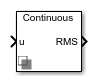# RMS Measurement

Calculate root-mean-square (RMS) properties of a signal

•Libraries:
Simscape / Electrical / Control / Measurements

## Description

The RMS Measurement block measures root-mean-square (RMS) properties of the input signal. You can use it to measure one of these properties:

• The total RMS of the input signal

• The RMS of the individual harmonics of the input signal that you specify.

Use the total RMS configuration with appropriate sensors to perform RMS voltage, current, or power analyses in your system.

You can use the harmonics configuration to perform total harmonic distortion analyses on systems with nonlinear loads such as:

• Converters

• Motor drives

• Inverters

### Equations

The total RMS value is calculated from the input signal xRMS as:

`${x}_{RMS}\left(t\right)=\sqrt{\frac{1}{T}{\int }_{t-T}^{t}x{\left(t\right)}^{2}dt},$`

where:

• T is the period of the input signal, or equivalently the inverse of its base frequency F.

• x is the input signal.

Because the calculation is performed over a period of time, the block requires T seconds to respond to a step change in the input signal. This condition also applies to startup.

The harmonic RMS component xk,RMS for harmonic k is calculated as:

`${x}_{k,RMS}\left(t\right)=G\left(\frac{2}{T}\right)\sqrt{{\left({\int }_{t-T}^{t}x\left(t\right)\mathrm{sin}\left(\frac{2\pi kt}{T}\right)dt\right)}^{2}+{\left({\int }_{t-T}^{t}x\left(t\right)\mathrm{cos}\left(\frac{2\pi kt}{T}\right)dt\right)}^{2}},$`

where G is equal to `0.5` for the DC component (k = 0) and $1/\sqrt{2}$ for the AC components (k > 0).

## Ports

### Input

expand all

Periodic input signal.

Data Types: `single` | `double`

### Output

expand all

Estimated RMS of the input signal. If you select Specify harmonics, the output is a vector with each element corresponding to a specified harmonic. Otherwise, the output is a scalar representing the total RMS.

Data Types: `single` | `double`

## Parameters

expand all

Base frequency of the input signal corresponding to the first harmonic.

Specify whether to output the total RMS of the input signals, or the individual harmonics that you specify.

Specify the harmonics for which to output an RMS.

#### Dependencies

To enable this parameter, select the Specify harmonics parameter.

Time between consecutive block executions. During execution, the block produces outputs and, if appropriate, updates its internal state. For more information, see What Is Sample Time? and Specify Sample Time.

For continuous operation, set this property to `0`. For discrete operation, specify the sample time explicitly as a positive number. This block does not support inherited sample time.

If this block is in a masked subsystem, or other variant subsystem that allows either continuous and discrete operation, promote the sample time parameter. Promoting the sample time parameter ensures correct switching between the continuous and discrete implementations of the block. For more information, see Promote Block Parameters on a Mask.

## Version History

Introduced in R2017b